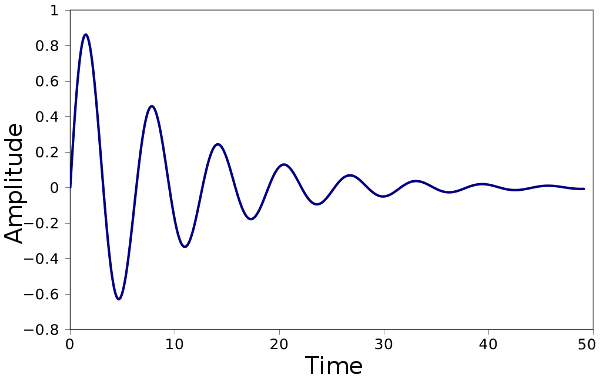### Graphene Resonators

June 24, 2011

If it wasn't for quantum mechanics, the universe would have wound down a long time ago. The essential idea of the quantum, that tiny things, like electrons in atoms, remain in a definite energy state until knocked really hard, is important, since macroscopic mechanics has one particular problem. A free oscillator, such a pendulum, has a lesser and lesser swing over time, and then it stops. A mechanism in a pendulum-based clock needs to push on the pendulum after each cycle to keep it going.

Friction forces in the pendulum pivot convert some of its motional energy to heat, so the harmonic oscillating motion of the pendulum is damped. The equation of motion of a damped harmonic oscillator is well developed and understood:
x(t) = e-δωot(A cos(ωdt) + A sin(ωdt))
where x(t) is the position at time t, ωo is the undamped oscillation frequency, δ is the damping coefficient, ωd is the damped oscillation frequency, and A and B are the initial conditions of motion; viz,
ωd = ωo sqrt(1 - δ2)
A = x(0)
B = (1/ωd)(δωox(0) + [dx/dt]0)
where x(0) is the position at time zero, [dx/dt]0 is the derivative of position evaluated at time zero, and the damping coefficient is between zero and one.

The plot below shows the amplitude of a damped harmonic oscillator having an undamped frequency ωo = 1 rad/sec, a damping coefficient of 0.1, an initial position of zero, and a derivative at zero of one sec-1. The damping causes a reduction in oscillating frequency to ωd = 0.995 rad/sec.Amplitude of a damped harmonic oscillator. See text for parameters. (Plot via Gnumeric)

As we reduce the size of resonators to nanoscale dimension, what factors affect the damping? A paper in Nature Communications lists the following factors:
1. Phonon–phonon interactions that cause thermoelastic damping.
2. Viscous or fluidic damping by the surrounding medium.
3. Material losses caused by the relaxation of bulk and surface defects.
4. Losses from the resonator supporting structure.

A team of researchers from the Quantum NanoElectronics Group at the Catalan Institute of Nanotechnology (Barcelona, Spain), and the Technische Universität München (Garching, Germany), found that interesting things happen when the mechanical resonator is shrunk as far as possible. The team fabricated nanoscale resonators of graphene sheets and carbon nanotubes by suspending them over grooves in a substrate and clamping the ends.[2-4]

Whereas the damping forces observed in resonators down to a few tens of nanometers in scale are linear, the team found that resonators of nanoscale graphene and carbon nanotubes show strong nonlinear damping forces. This non-linearity allowed fabrication of resonators with a high quality ("Q") factor. They were able to make a graphene resonator with a Q of 100,000, a new record.

Since these resonators have high Q-factor and low mass, they would make excellent force and mass sensors. The addition of even a small mass, such as an analyte bonding to a sensitized surface, would cause a large change in the resonance frequency.

### References:

Linked Keywords: Quantum mechanics; universe; electron; atom; energy level; energy state; macroscopic mechanics; oscillator; pendulum; clock; friction forces; heat; harmonic oscillator; damped harmonic oscillator; amplitude; radian per second; rad/sec; Gnumeric; nanoscale; Nature Communications; phonon; thermoelastic damping; viscosity; fluidic; crystallographic defect; Quantum NanoElectronics Group; Catalan Institute of Nanotechnology; Barcelona, Spain; Technical University Munich; Technische Universität München; Garching bei München; Garching, Germany; graphene sheet; carbon nanotube; linear; nonlinear; Q-factor; quality factor; sensor; analyte.